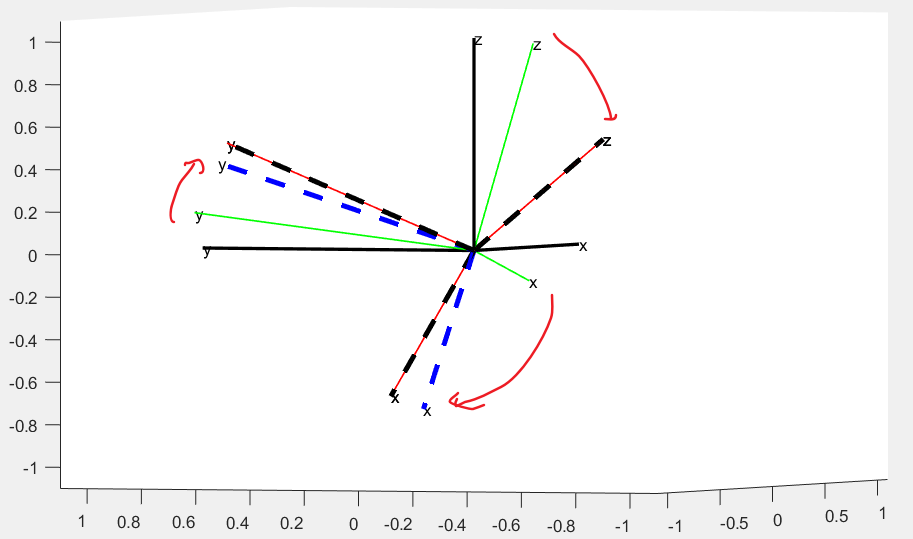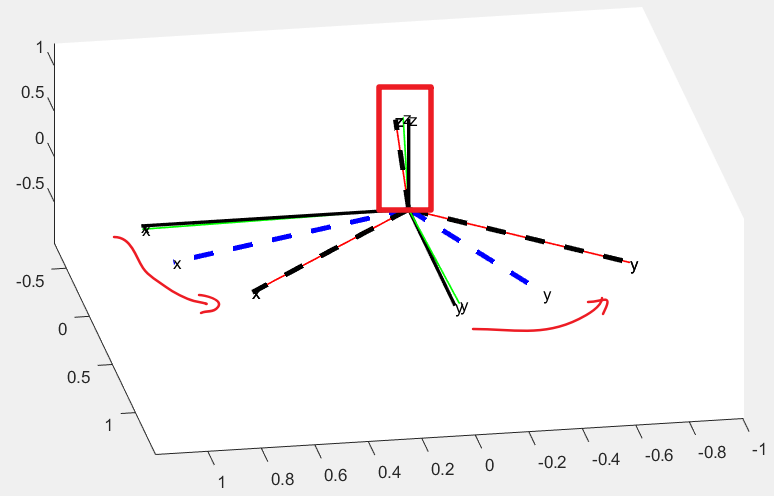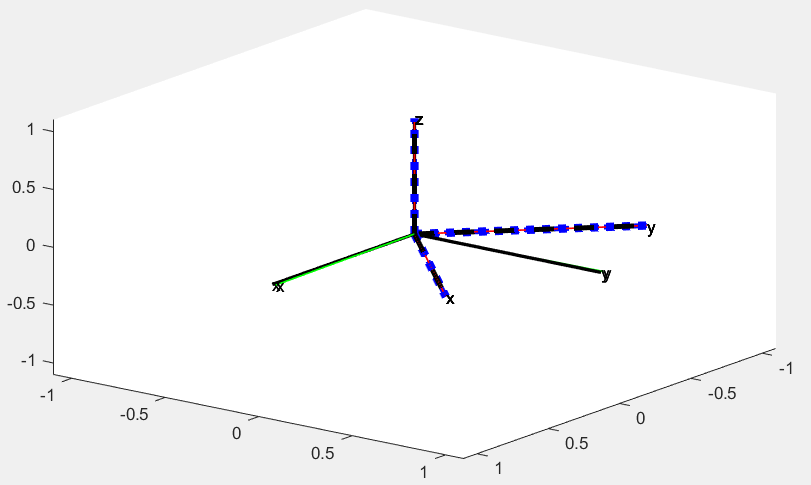# 【飞控】倾转分离 (二)-PX4 倾转分离，效果验证

1.如果同时3个轴误差都很大那么，结果会对齐z轴 ，即没有roll 和 pitch 误差，但是会有一定的 yaw 的误差。

2.因为使用的是固定衰减系数，只要roll 和 pitch 的误差不等于0，那么 yaw 的误差就会被保留。

(相比比之前这样roll,pitch的优先级被再次提高，以前是变化的衰减系数，只有保证roll,pitch的误差比较小即可，现在是必须没有误差，才去控制yaw)global x_axis_last;
global y_axis_last;
global z_axis_last;
x_axis_last=[1,0,0];
y_axis_last=[0,1,0];
z_axis_last=[0,0,1];
NED=[0 0 0];
Cur_angle=[10 10 10];
Des_angle=[45 45 45];
%根据 roll pitch yaw 的外环增益 计算 权重
attitude_gain =[6.5 , 6.5 , 2.8];
roll_pitch_gain = (attitude_gain(1) + attitude_gain(2)) / 2.0;
yaw_w = constrain_float(attitude_gain(3) / roll_pitch_gain, 0.0, 1.0)
attitude_gain(3) = roll_pitch_gain;

%弧度

%这个画图画的是在以N系为基础的旋转 即 地理坐标系的旋转
plot_body_cur_axis_in_NED([0,0,0],NED,['k','k','k'],'-',2)
plot_body_cur_axis_in_NED([0,0,0],Cur_angle,['g','g','g'],'-',1)
plot_body_cur_axis_in_NED([0,0,0],Des_angle,['r','r','r'],'-',1)

%当前姿态 Ccur->N
q=Q_normalize(q);
%当前姿态的旋转矩阵 取出z轴列（z轴单位向量） Zcur(N;)
e_z=cur_dcm*[0;0;1];
%期望姿态 Ctar->N
qd=Q_normalize(qd);
%期望姿态的旋转矩阵 取出z轴列（z轴单位向量） Ztar(N)
e_z_d=des_dcm*[0;0;1];

%PX4_Q（当前,期望） 得到的是 当前->期望的旋转 Q cur-> tb1
qd_red=PX4_Q(e_z,e_z_d);%%增量 可以加在任何坐标系下 可以等同于机体坐标系下想旋转

if (abs(qd_red(1)) > (1 - 1e-5) || abs(qd_red(2)) > (1 - 1e-5))
qd_red = qd;
else
%把转轴 转到N系
%q(cb->n)^-1 qd_red q(cb->n)
%把 误差 转到 N系
%q(cb->n) *  q(cb->n)^-1 qd_red q(cb->n)  = qd_red q(cb->n)
%得到地理系下的误差 Qtb1-N
qd_red = Q_multipli(qd_red , q);  %
% 画出Qtb1在地理系下的坐标
%plot_body_tar_axis_in_NED_Q([0,0,0],NED ,qd_red ,['c','c','c'],':',5)
end

%Qtar->tb1 = Qtb1->n^-1 * Qtar->n
q_mix = Q_multipli( Q_INV(qd_red) , qd );
% 画出 目标 位置 减去 旋转误差 在地理系下的坐标
%plot_body_tar_axis_in_NED_Q([0,0,0],Des_angle ,Q_INV(q_mix) ,['r','r','r'],'--',3)

%优化旋转误差
q_mix = q_mix*signNoZero(q_mix(1));
q_mix(1) = constrain_float(q_mix(1), -1, 1);
q_mix(4) = constrain_float(q_mix(4), -1, 1);

%限制旋转误差  组合成新的旋转 qd= Qtb1->N * Qtar->tb1 = Qtar->N
qd = Q_multipli( qd_red , [cos(yaw_w * acos(q_mix(1))), 0, 0, sin(yaw_w * asin(q_mix(4)))]);

%新的 Qtar->cur
qe = Q_multipli( Q_INV(q) , qd) ;

plot_body_tar_axis_in_NED_Q([0,0,0],Cur_angle ,qe ,['b','b','b'],'--',3) 

ok，今天就讲这么多，我是zing,一个有趣的算法工程师，我们下期见。